What does it do?

It returns a value that you set if a condition is met, and a value if it is not met

Formula breakdown:

=IF(Logical Test,Value if True,Value if False)

What it means:

=IF(The condition to be checked, Value to be shown if the condition is met, Value to be shown if condition is not met)

The IF function is probably one of the most used Excel functions because it is easy to understand and very flexible when you apply it to real life situations.

Here I will show you a couple of ways that you can use the IF function to get you up and going.We want to show a Bonus value if sales are bigger than \$3000, and No Bonus is shown if this condition is not met. Afterwards let’s try computing the 10% bonus!

STEP 1: We need to enter the IF function in a blank cell:

## =IF(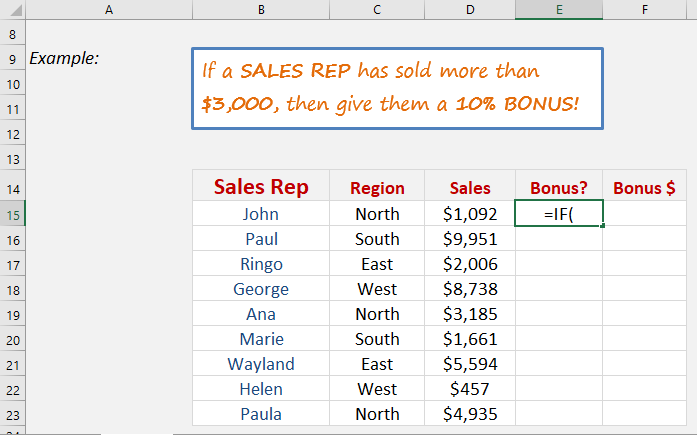STEP 2: The IF arguments:

## Logical_test

Sales Rep has sold more than 3000 dollars.

## =IF(D15>3000,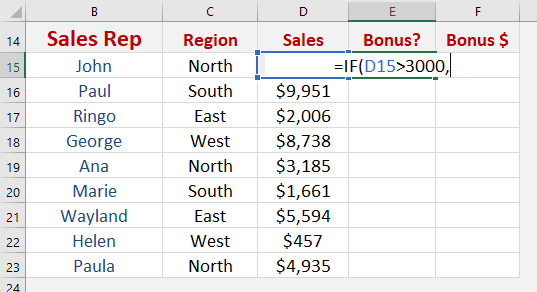## Value_if_true

What value should be displayed if the condition is true?

We want “Bonus” to be displayed

## =IF(D15>3000, “Bonus”,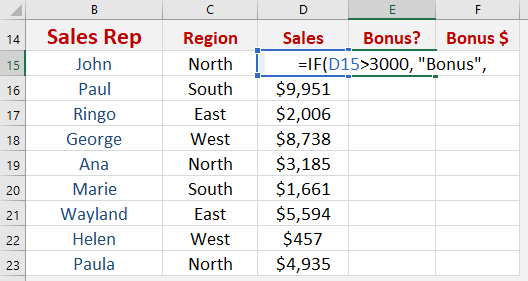## Value_if_false

What value should be displayed if the condition is false?

We want “No Bonus” to be displayed

## =IF(D15>3000, “Bonus”, “No Bonus”)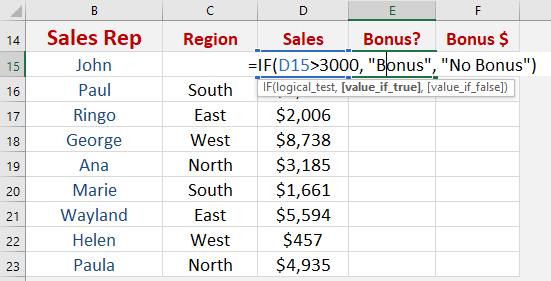Apply the same formula to the rest of the cells by dragging the lower right corner downwards.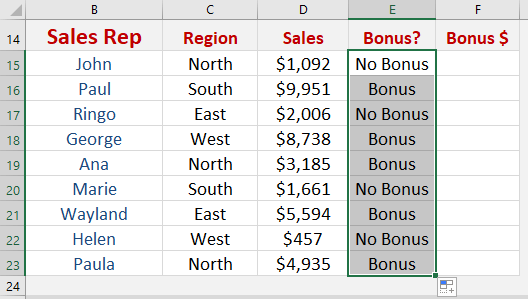STEP 3: Let us now aim to give the 10% Bonus!

The IF arguments:

## Logical_test

Sales Rep has sold more than 3000 dollars.

## =IF(D15>3000,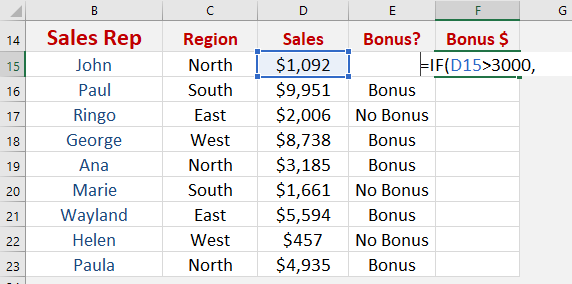## Value_if_true

What value should be displayed if the condition is true?

We want give a 10% bonus based on sales

## =IF(D15>3000, D15*10%,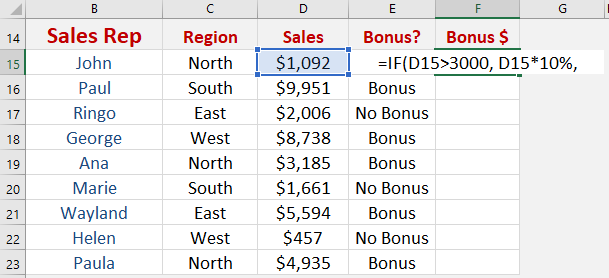## Value_if_false

What value should be displayed if the condition is false?

Then no bonus amount should be given, type in 0

## =IF(D15>3000, D15*10%, 0)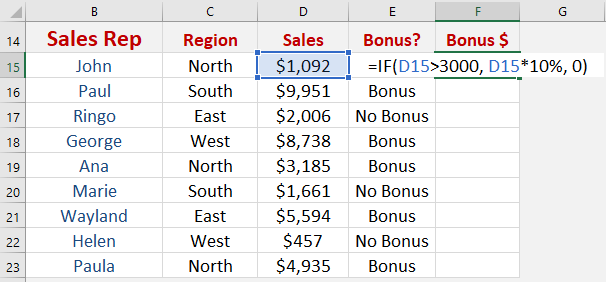Apply the same formula to the rest of the cells by dragging the lower right corner downwards.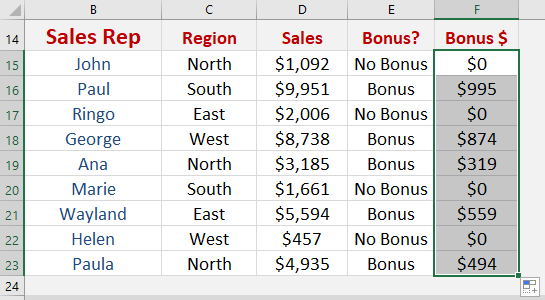You now have all of results!

How to Use the If Formula in Excel